# Leaky Joins

### Ying Yang, Oliver Kennedy### Disclaimer

Ying could not be here today. If you like her ideas, get in touch with her.

(If you don't, blame my presentation)

(Also, Ying is on the job market)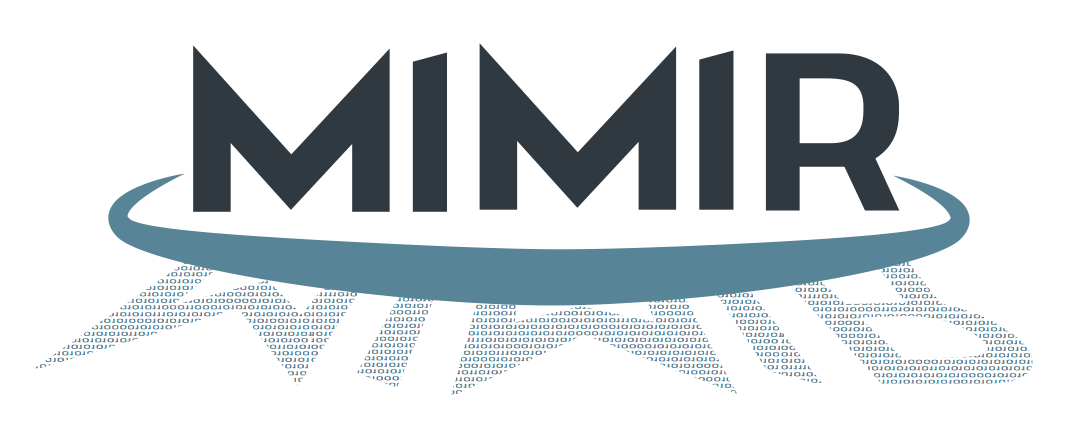http://mimirdb.info

(not immediately relevant to the talk, but you should check it out)

## Roughly 1-2 years ago...

Ying: To implement {cool feature in Mimir}, we'll need to be able to perform inference on Graphical Models, but we will not know how complex they are.

## Graphical Models

Joint probability distributions are expensive to store
$$p(D, I, G, S, J)$$

Bayes rule lets us break apart the distribution
$$= p(D, I, G, S) \cdot p(J | D, I, G, S)$$

And conditional independence lets us further simplify
$$= p(D, I, G, S) \cdot p(J | G, S)$$

This is basis for a type of graphical model called a "Bayes Net"

## Bayesean Networks

$p(D=1, I=0, S=0, G=2, J=1)$
$=\;0.5$ $\cdot\;0.7$ $\cdot\;0.95$ $\cdot\;0.25$ $\cdot\;0.8$ $=\;0.0665$

$p(D,I,S,G,J)$
$=$
$p(D) \bowtie p(I) \bowtie p(S|I) \bowtie p(G|D,I) \bowtie p(J|G,S)$

## Inference

$p(J) = \sum_{D,I,S,G}p(D,I,S,G,J)$

(aka the computing the marginal probability)

## Inference Algorithms

Exact (e.g. Variable Elimination)
Fast and precise, but scales poorly with graph complexity.
Approximate (e.g. Gibbs Sampling)
Consistent performance, but only asymptotic convergence.

Key Challenge: For {really cool feature} we don't know whether we should use exact or approximate inference.

Can we gracefully degrade from exact to approximate inference?


SELECT J.J, SUM(D.p * I.p * S.p * G.p * J.p) AS p
FROM D NATURAL JOIN I NATURAL JOIN S
NATURAL JOIN G NATURAL JOIN J
GROUP BY J.J


(Inference is essentially a big group-by aggregate join query)

(Variable elimination is Aggregate Pushdown + Join Ordering)

## Online Aggregation (OLA)

$Avg(3,6,10,9,1,3,9,7,9,4,7,9,2,1,2,4,10,8,9,7) = 6$

$Avg(3,6,10,9,1) = 5.8$ $\approx 6$

$Sum\left(\frac{k}{N} Samples\right) \cdot \frac{N}{k} \approx Sum(*)$

Sampling lets you approximate aggregate values with orders of magnitude less data.

## Typical OLA Challenges

$Sample(R) \bowtie Sample(S)$ is likely to be empty.
Stratified Sampling
It doesn't matter how important they are to the aggregate, rare samples are still rare.
Replacement
Does the sampling algorithm converge exactly or asymptotically?

## Replacement

Sampling Without Replacement
... eventually converges to a precise answer.
Sampling With Replacement
... doesn't need to track what's been sampled.
... produces a better behaved estimate distribution.

## OLA over GMs

Tables are Small
Compute, not IO is the bottleneck.
Tables are Dense
Birthday Paradox and Stratified Sampling irrelevant.
Queries have High Tree-Width
Intermediate tables are large.

Classical OLA techniques aren't entirely appropriate.

## A Few Quick Insights

2. Dense Tables mean we can sample directly from join outputs.
3. Cyclic PRNGs like Linear Congruential Generators can be used to generate a randomly ordered, but non-repeating sequence of integers from $0$ to any $N$ in constant memory.

## Linear Congruential Generators

If you pick $a$, $b$, and $N$ correctly, then the sequence:

$K_i = (a\cdot K_{i−1}+b)\;mod\;N$

will produce $N$ distinct, pseudorandom integers $K_i \in [0, N)$

## Cyclic Sampling

To marginalize $p(\{X_i\})$...

1. Init an LCG with a cycle of $N = \prod_i |dom(X_i)|$
2. Use the LCG to sample $\{x_i\} \in \{X_i\}$
3. Incorporate $p(x_i = X_i)$ into the OLA estimate
4. Repeat from 2 until done

## Accuracy

Sampling with Replacement
Chernoff Bounds, Hoeffding Bounds give an $\epsilon-\delta$ guarantee on the sum/avg of a sample with replacement.
Without Replacement?
Serfling et. al. have a variant of Hoeffding Bounds for sampling without replacement.

## Cyclic Sampling

Progressively better estimates over time.
Converges in bounded time.
Exponential time in the number of variables.

## Better OLA: Leaky Joins

Make Cyclic Sampling into a composable operator$G$#$\sum p_{\psi_2}$
110.126

$I$$G#\sum p_{\psi_1} 0110.18G#\sum p_{\psi_2} 130.348 240.288 340.350 I$$G$#$\sum p_{\psi_1}$
0120.140
1120.222
0220.238
1220.050
0320.322
1320.028$G$#$\sum p_{\psi_2}$
140.362
240.288
340.350
$I$$G$#$\sum p_{\psi_1}$
0120.140
1120.222
0220.238
1220.050
0320.322
1320.028

## Leaky Joins

1. Build a normal join/aggregate graph as in variable elimination: One Cyclic Sampler for each Join+Aggregate.
2. Keep advancing Cyclic Samplers in parallel, resetting their output after every cycle so samples "leak" through.
3. When the sampler completes one full cycle with a complete input, mark it complete and stop sampling it.
4. Continue until a desired accuracy is reached or all tables marked complete.

There's a bit of extra math to compute $\epsilon-\delta$ bounds by adapting Serfling's results. It's in the paper.

## Experiments

Microbenchmarks
Fix time, vary domain size, measure accuracy
Fix domain size, vary time, measure accuracy
Vary domain size, measure time to completion
Macrobenchmarks
4 graphs from the bnlearn Repository

## Microbenchmarks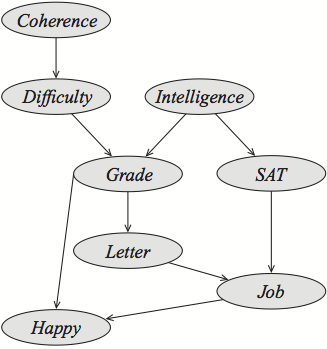Student: A common benchmark graph.

## Accuracy vs Domain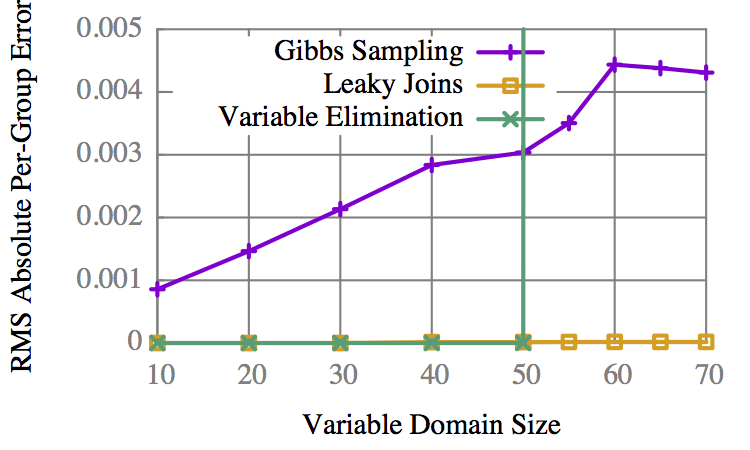VE is binary: It completes, or it doesn't.

## Accuracy vs Time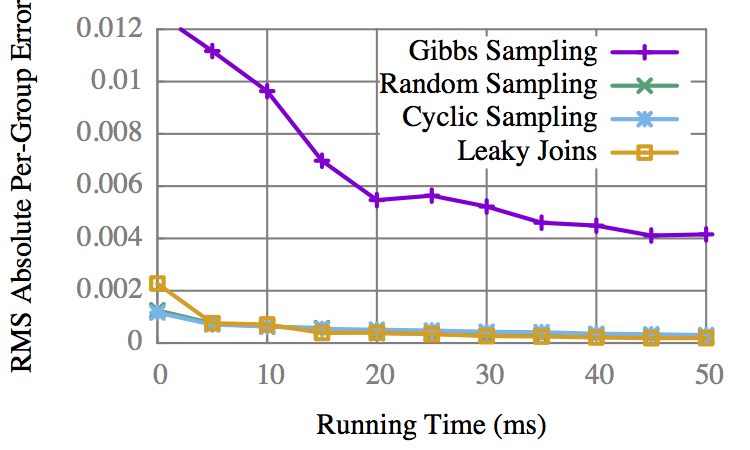CS gets early results faster, but is overtaken by LJ.

## Domain vs Time to 100%LJ is only 3-5x slower than VE.

## "Child"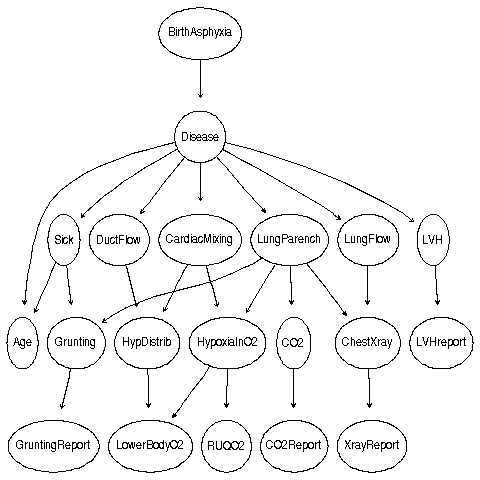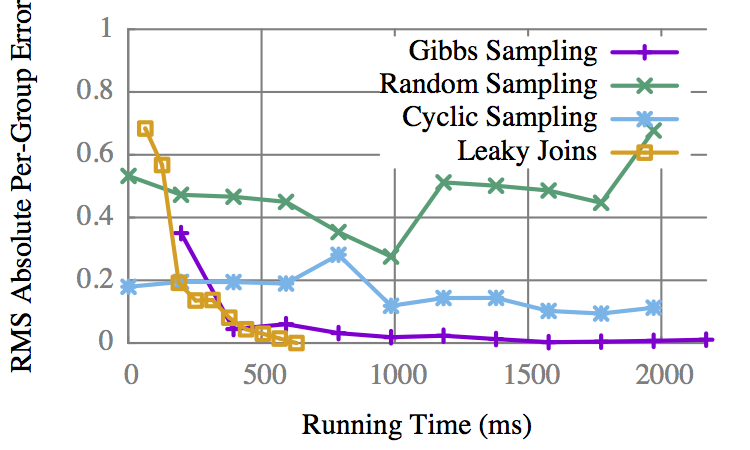LJ converges to an exact result before Gibbs gets an approx.

## "Insurance"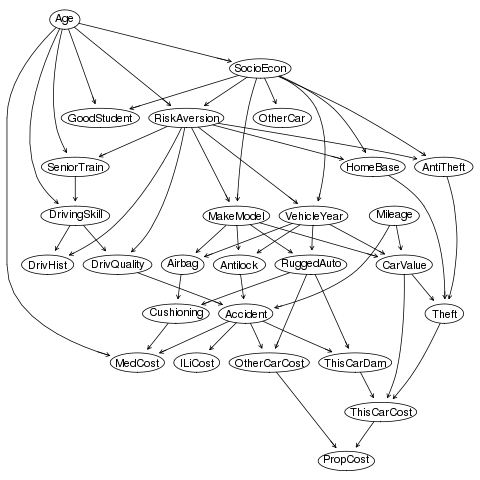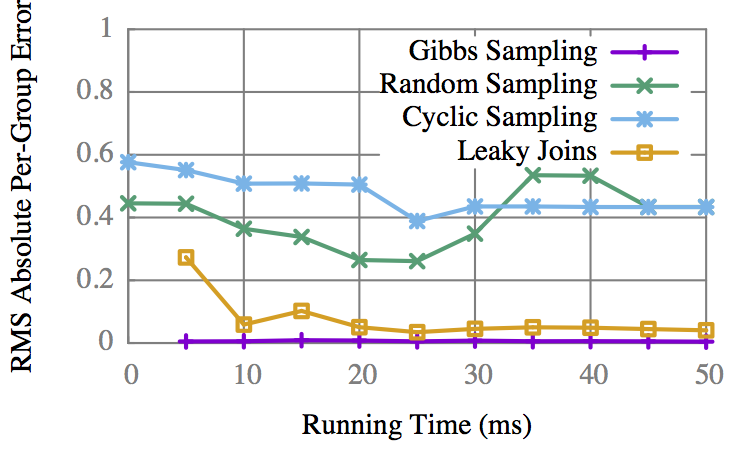On some graphs Gibbs is better, but only marginally.

## Leaky Joins

• Classical OLA isn't appropriate for GMs.
• Idea 1: LCGs can sample without replacement.
• Idea 2: "Leak" samples through a normal join graph.
• Compared to both Variable Elim. and Gibbs Sampling, Leaky Joins are often better and never drastically worse.

Questions?